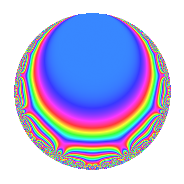# Properties

 Label 804.2.o.cLevel 804 Weight 2 Character orbit 804.o Analytic conductor 6.420 Analytic rank 0 Dimension 4 CM No Inner twists 2

# Related objects

## Newspace parameters

 Level: $$N$$ = $$804 = 2^{2} \cdot 3 \cdot 67$$ Weight: $$k$$ = $$2$$ Character orbit: $$[\chi]$$ = 804.o (of order $$6$$ and degree $$2$$)

## Newform invariants

 Self dual: No Analytic conductor: $$6.41997232251$$ Analytic rank: $$0$$ Dimension: $$4$$ Relative dimension: $$2$$ over $$\Q(\zeta_{6})$$ Coefficient field: $$\Q(\sqrt{-2}, \sqrt{-3})$$ Coefficient ring: $$\Z[a_1, \ldots, a_{17}]$$ Coefficient ring index: $$1$$ Sato-Tate group: $\mathrm{SU}(2)[C_{6}]$

## $q$-expansion

Coefficients of the $$q$$-expansion are expressed in terms of a basis $$1,\beta_1,\beta_2,\beta_3$$ for the coefficient ring described below. We also show the integral $$q$$-expansion of the trace form.

 $$f(q)$$ $$=$$ $$q + ( 1 + \beta_{3} ) q^{3} -2 q^{5} + ( 2 - 2 \beta_{1} - \beta_{2} + 2 \beta_{3} ) q^{7} + ( -1 + 2 \beta_{3} ) q^{9} +O(q^{10})$$ $$q + ( 1 + \beta_{3} ) q^{3} -2 q^{5} + ( 2 - 2 \beta_{1} - \beta_{2} + 2 \beta_{3} ) q^{7} + ( -1 + 2 \beta_{3} ) q^{9} + ( \beta_{1} - \beta_{2} + \beta_{3} ) q^{11} + ( 3 + 3 \beta_{2} ) q^{13} + ( -2 - 2 \beta_{3} ) q^{15} + ( -1 - 3 \beta_{1} - \beta_{2} ) q^{17} + ( -3 + 3 \beta_{2} ) q^{19} + ( 2 - \beta_{1} - 5 \beta_{2} + 3 \beta_{3} ) q^{21} + ( -3 + 3 \beta_{1} - 3 \beta_{2} ) q^{23} - q^{25} + ( -5 + \beta_{3} ) q^{27} + ( 2 - 5 \beta_{1} - \beta_{2} + 5 \beta_{3} ) q^{29} + ( -2 + \beta_{2} ) q^{31} + ( -4 + 2 \beta_{1} + \beta_{2} ) q^{33} + ( -4 + 4 \beta_{1} + 2 \beta_{2} - 4 \beta_{3} ) q^{35} + ( -5 - 2 \beta_{1} + 5 \beta_{2} + 4 \beta_{3} ) q^{37} + ( 3 - 3 \beta_{1} + 3 \beta_{2} + 6 \beta_{3} ) q^{39} + ( -\beta_{1} - \beta_{2} - \beta_{3} ) q^{41} + ( 4 - 8 \beta_{2} + 4 \beta_{3} ) q^{43} + ( 2 - 4 \beta_{3} ) q^{45} + ( -2 + \beta_{1} + \beta_{2} - \beta_{3} ) q^{47} + ( 4 - 4 \beta_{1} - 4 \beta_{2} + 8 \beta_{3} ) q^{49} + ( 5 - 2 \beta_{1} - 7 \beta_{2} - 2 \beta_{3} ) q^{51} + 6 q^{53} + ( -2 \beta_{1} + 2 \beta_{2} - 2 \beta_{3} ) q^{55} + ( -3 - 3 \beta_{1} + 3 \beta_{2} ) q^{57} + ( -2 + 4 \beta_{2} - 2 \beta_{3} ) q^{59} + ( 3 + 4 \beta_{1} + 3 \beta_{2} ) q^{61} + ( -2 + 4 \beta_{1} - 7 \beta_{2} ) q^{63} + ( -6 - 6 \beta_{2} ) q^{65} + ( 9 - 2 \beta_{2} ) q^{67} + ( -9 + 6 \beta_{1} + 3 \beta_{2} - 6 \beta_{3} ) q^{69} + ( 10 + 3 \beta_{1} - 5 \beta_{2} - 3 \beta_{3} ) q^{71} + ( 1 + 2 \beta_{1} - \beta_{2} - 4 \beta_{3} ) q^{73} + ( -1 - \beta_{3} ) q^{75} + ( -5 + 5 \beta_{1} - 5 \beta_{2} ) q^{77} + ( -6 + 4 \beta_{1} + 3 \beta_{2} - 4 \beta_{3} ) q^{79} + ( -7 - 4 \beta_{3} ) q^{81} + ( -1 - 7 \beta_{1} - \beta_{2} ) q^{83} + ( 2 + 6 \beta_{1} + 2 \beta_{2} ) q^{85} + ( 2 - 4 \beta_{1} - 11 \beta_{2} + 6 \beta_{3} ) q^{87} + ( -6 + 12 \beta_{2} - 4 \beta_{3} ) q^{89} + ( 9 - 12 \beta_{1} + 6 \beta_{3} ) q^{91} + ( -2 - \beta_{1} + \beta_{2} - \beta_{3} ) q^{93} + ( 6 - 6 \beta_{2} ) q^{95} + ( 3 + 6 \beta_{1} + 3 \beta_{2} ) q^{97} + ( -8 + \beta_{1} + 5 \beta_{2} - 3 \beta_{3} ) q^{99} +O(q^{100})$$ $$\operatorname{Tr}(f)(q)$$ $$=$$ $$4q + 4q^{3} - 8q^{5} + 6q^{7} - 4q^{9} + O(q^{10})$$ $$4q + 4q^{3} - 8q^{5} + 6q^{7} - 4q^{9} - 2q^{11} + 18q^{13} - 8q^{15} - 6q^{17} - 6q^{19} - 2q^{21} - 18q^{23} - 4q^{25} - 20q^{27} + 6q^{29} - 6q^{31} - 14q^{33} - 12q^{35} - 10q^{37} + 18q^{39} - 2q^{41} + 8q^{45} - 6q^{47} + 8q^{49} + 6q^{51} + 24q^{53} + 4q^{55} - 6q^{57} + 18q^{61} - 22q^{63} - 36q^{65} + 32q^{67} - 30q^{69} + 30q^{71} + 2q^{73} - 4q^{75} - 30q^{77} - 18q^{79} - 28q^{81} - 6q^{83} + 12q^{85} - 14q^{87} + 36q^{91} - 6q^{93} + 12q^{95} + 18q^{97} - 22q^{99} + O(q^{100})$$

Basis of coefficient ring in terms of a root $$\nu$$ of $$x^{4} - 2 x^{2} + 4$$:

 $$\beta_{0}$$ $$=$$ $$1$$ $$\beta_{1}$$ $$=$$ $$\nu$$ $$\beta_{2}$$ $$=$$ $$\nu^{2}$$$$/2$$ $$\beta_{3}$$ $$=$$ $$\nu^{3}$$$$/2$$
 $$1$$ $$=$$ $$\beta_0$$ $$\nu$$ $$=$$ $$\beta_{1}$$ $$\nu^{2}$$ $$=$$ $$2 \beta_{2}$$ $$\nu^{3}$$ $$=$$ $$2 \beta_{3}$$

## Character Values

We give the values of $$\chi$$ on generators for $$\left(\mathbb{Z}/804\mathbb{Z}\right)^\times$$.

 $$n$$ $$269$$ $$337$$ $$403$$ $$\chi(n)$$ $$-1$$ $$\beta_{2}$$ $$1$$

## Embeddings

For each embedding $$\iota_m$$ of the coefficient field, the values $$\iota_m(a_n)$$ are shown below.

For more information on an embedded modular form you can click on its label.

Label $$\iota_m(\nu)$$ $$a_{2}$$ $$a_{3}$$ $$a_{4}$$ $$a_{5}$$ $$a_{6}$$ $$a_{7}$$ $$a_{8}$$ $$a_{9}$$ $$a_{10}$$
365.1
 1.22474 − 0.707107i −1.22474 + 0.707107i −1.22474 − 0.707107i 1.22474 + 0.707107i
0 1.00000 1.41421i 0 −2.00000 0 −0.949490 0.548188i 0 −1.00000 2.82843i 0
365.2 0 1.00000 + 1.41421i 0 −2.00000 0 3.94949 + 2.28024i 0 −1.00000 + 2.82843i 0
641.1 0 1.00000 1.41421i 0 −2.00000 0 3.94949 2.28024i 0 −1.00000 2.82843i 0
641.2 0 1.00000 + 1.41421i 0 −2.00000 0 −0.949490 + 0.548188i 0 −1.00000 + 2.82843i 0
 $$n$$: e.g. 2-40 or 990-1000 Significant digits: Format: Complex embeddings Normalized embeddings Satake parameters Satake angles

## Inner twists

Char. orbit Parity Mult. Self Twist Proved
1.a Even 1 trivial yes
201.f Even 1 yes

## Hecke kernels

This newform can be constructed as the kernel of the linear operator $$T_{5} + 2$$ acting on $$S_{2}^{\mathrm{new}}(804, [\chi])$$.